# RS Aggarwal Solutions for Class 10 Maths Chapter 7 Triangles Exercise 7A

R S Aggarwal Solutions for class 10 Maths chapter 7 PDF equip you with answers to the questions in the textbook. All solutions for class 10 exercise 7A has been prepared by subject experts according to the latest CBSE syllabus. These solutions are available in PDF format and students can freely download it from our website.

## Download PDF of RS Aggarwal Solutions for Class 10 Maths Chapter 7 Triangles Exercise 7A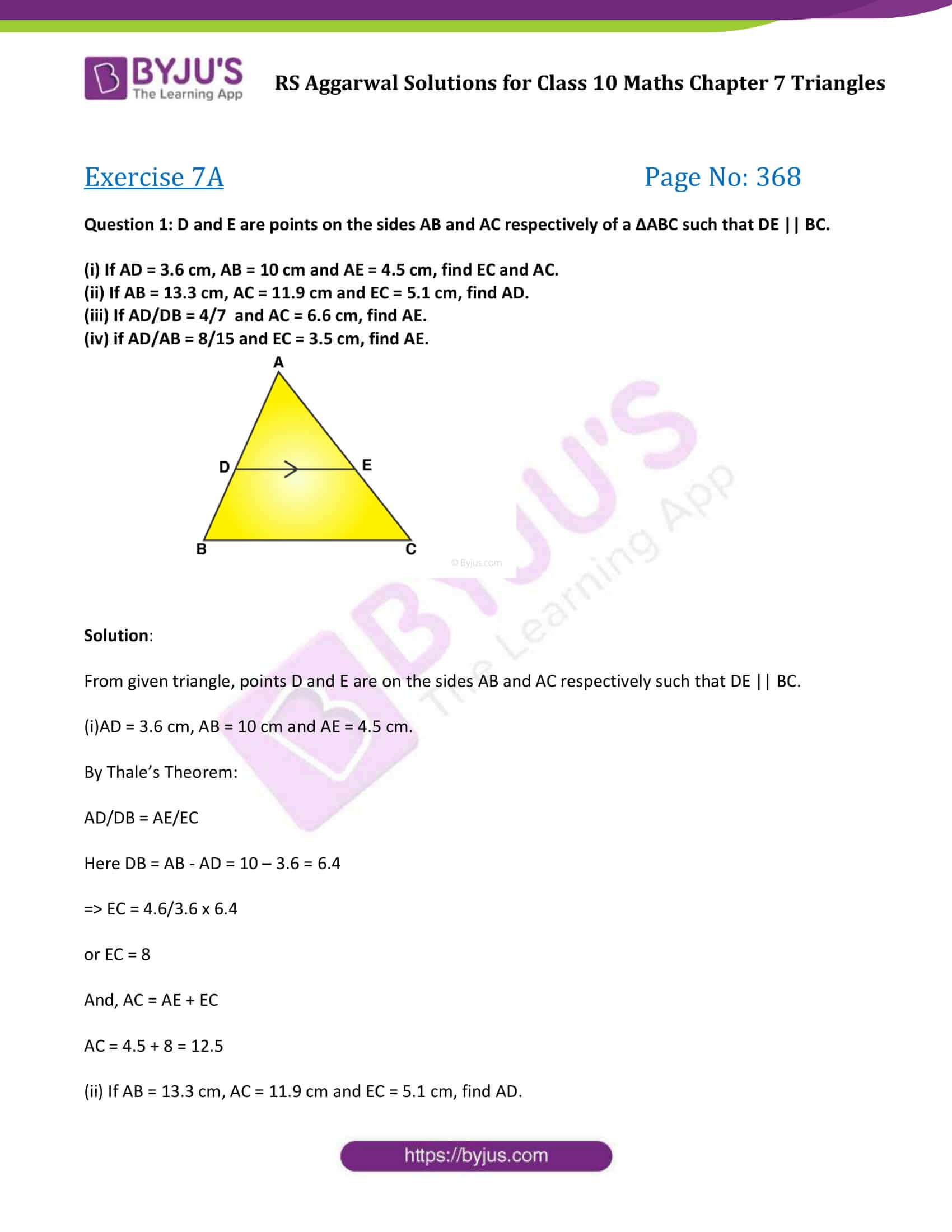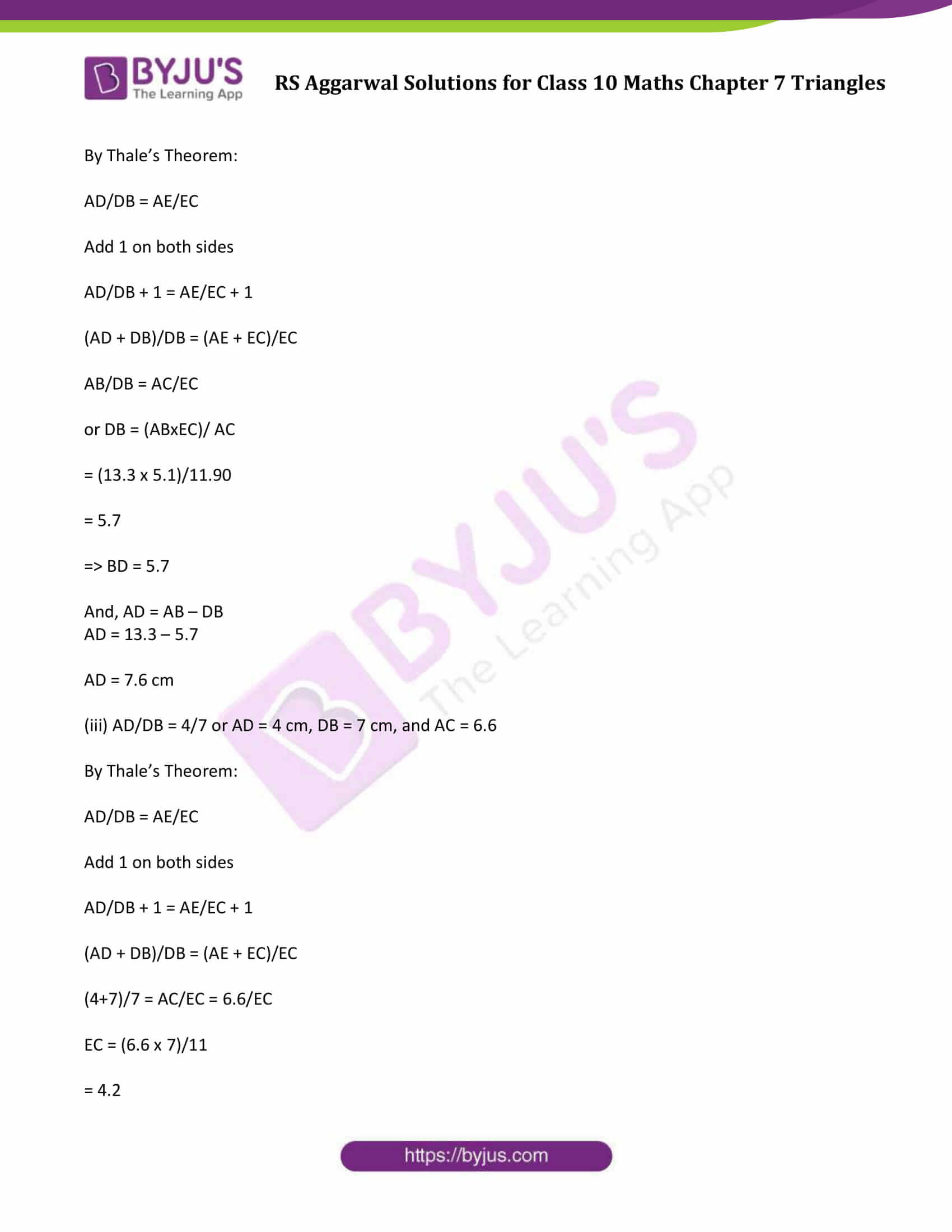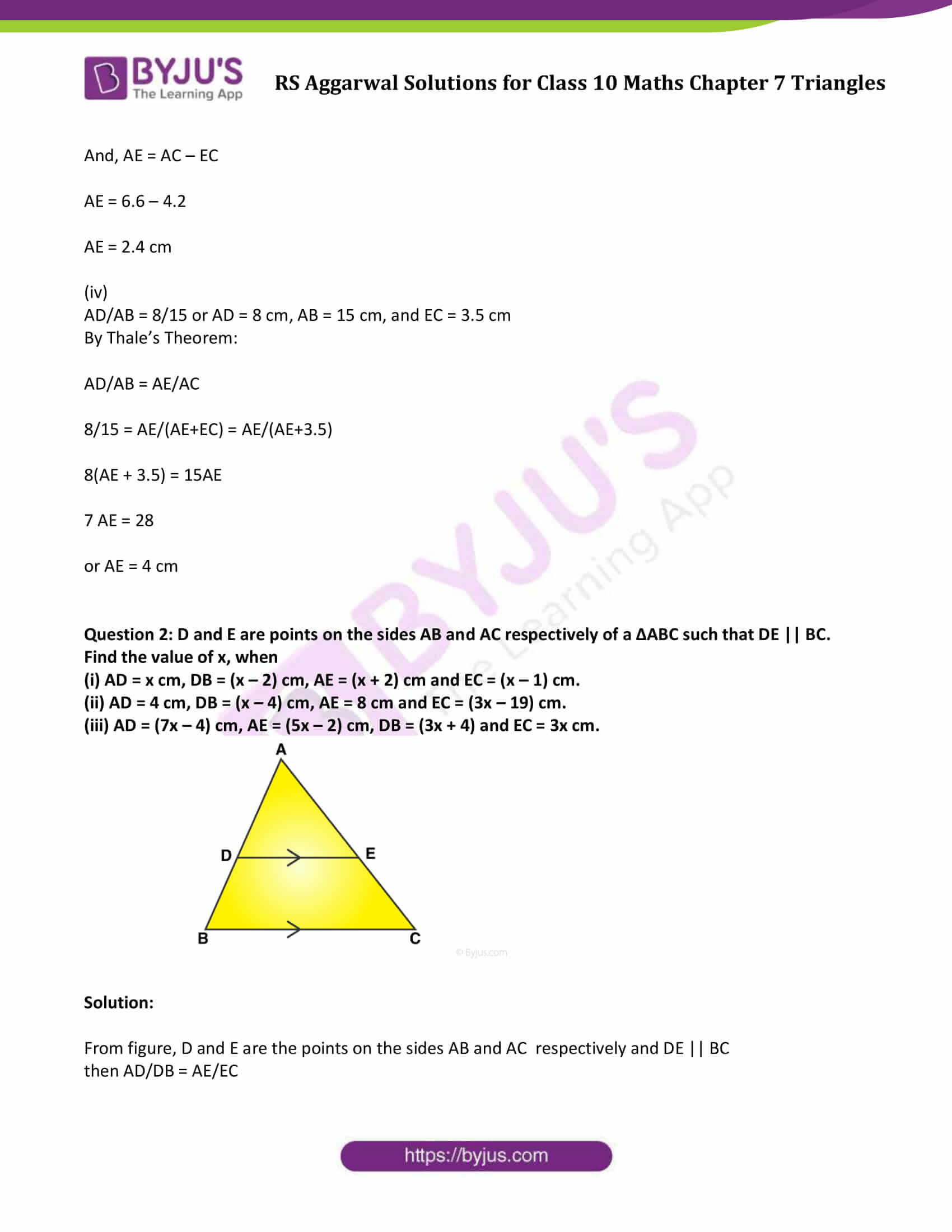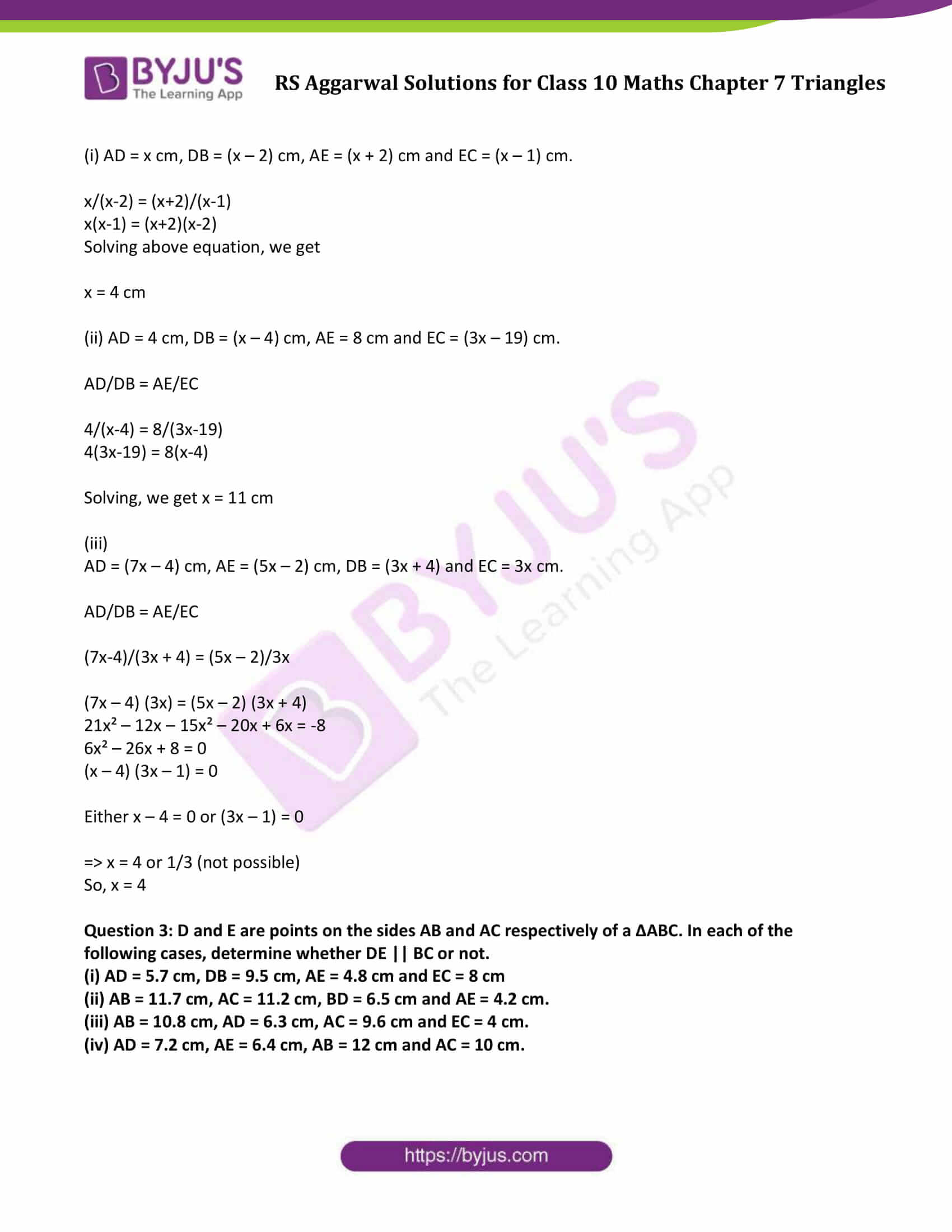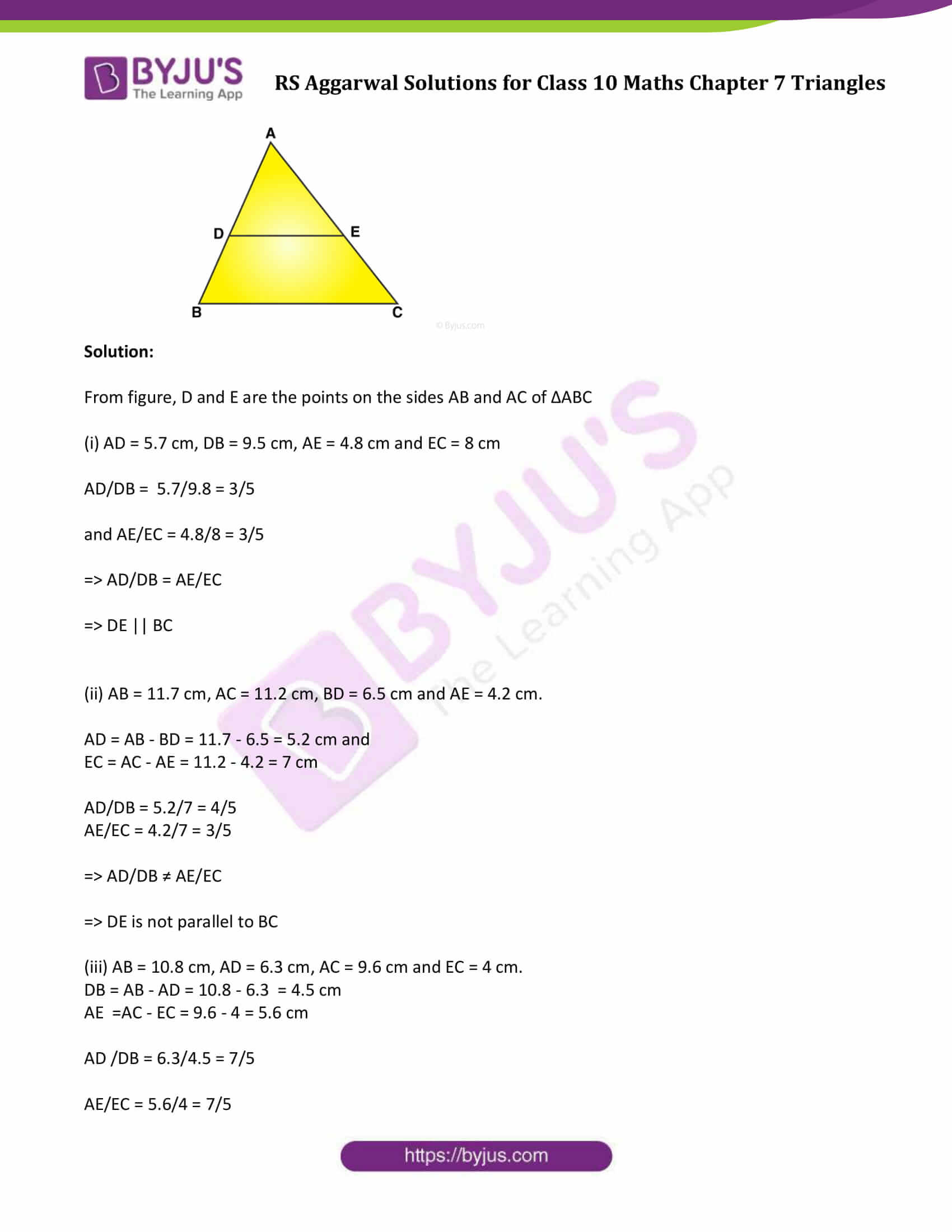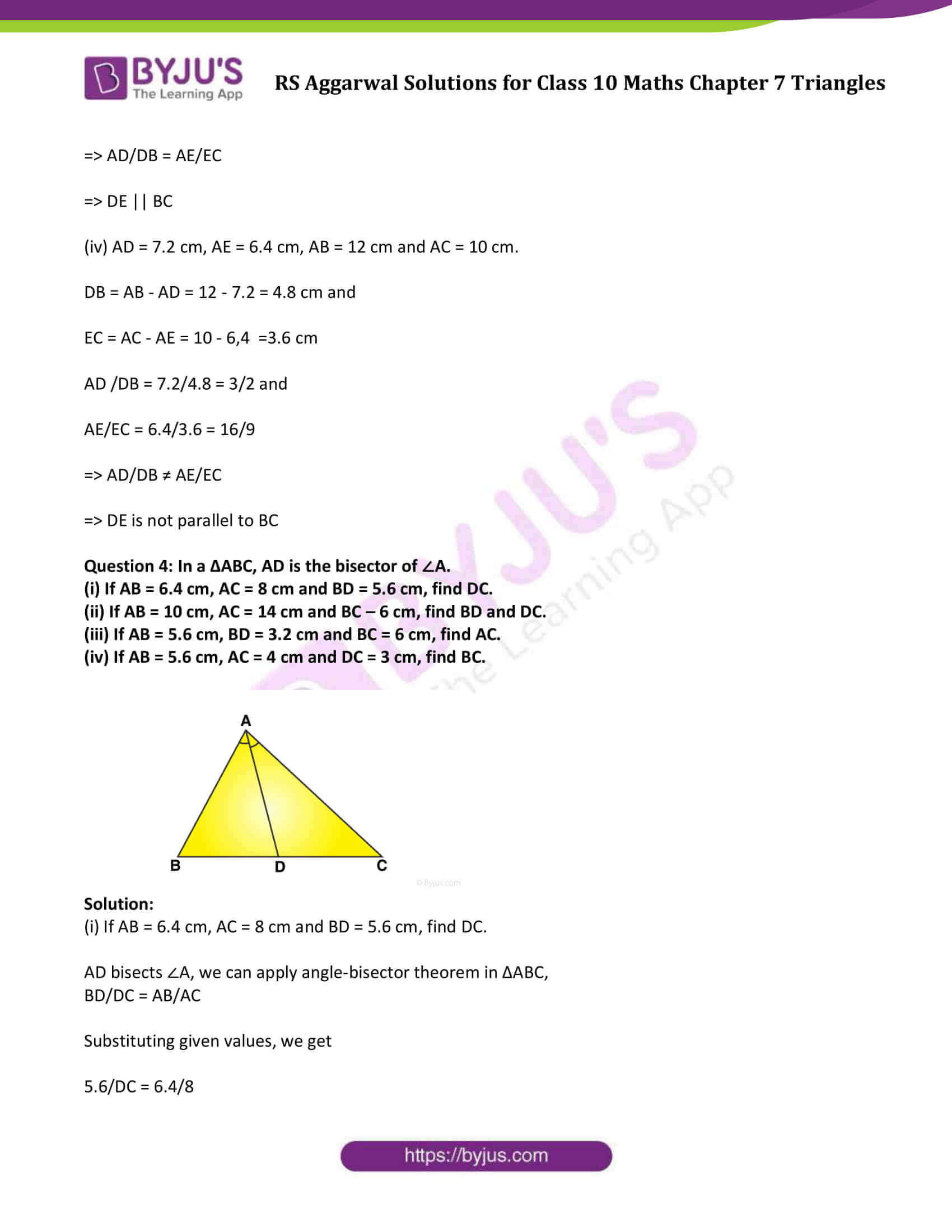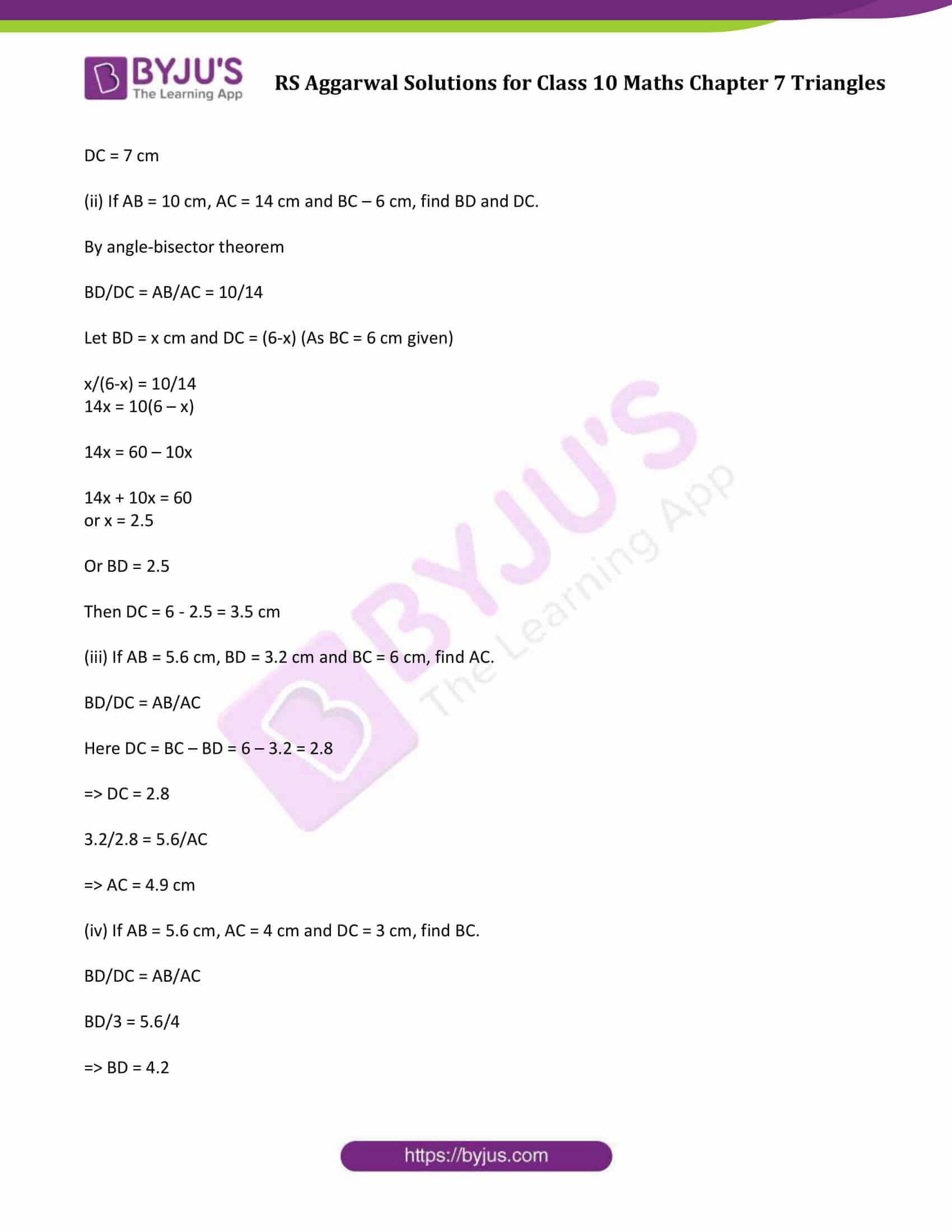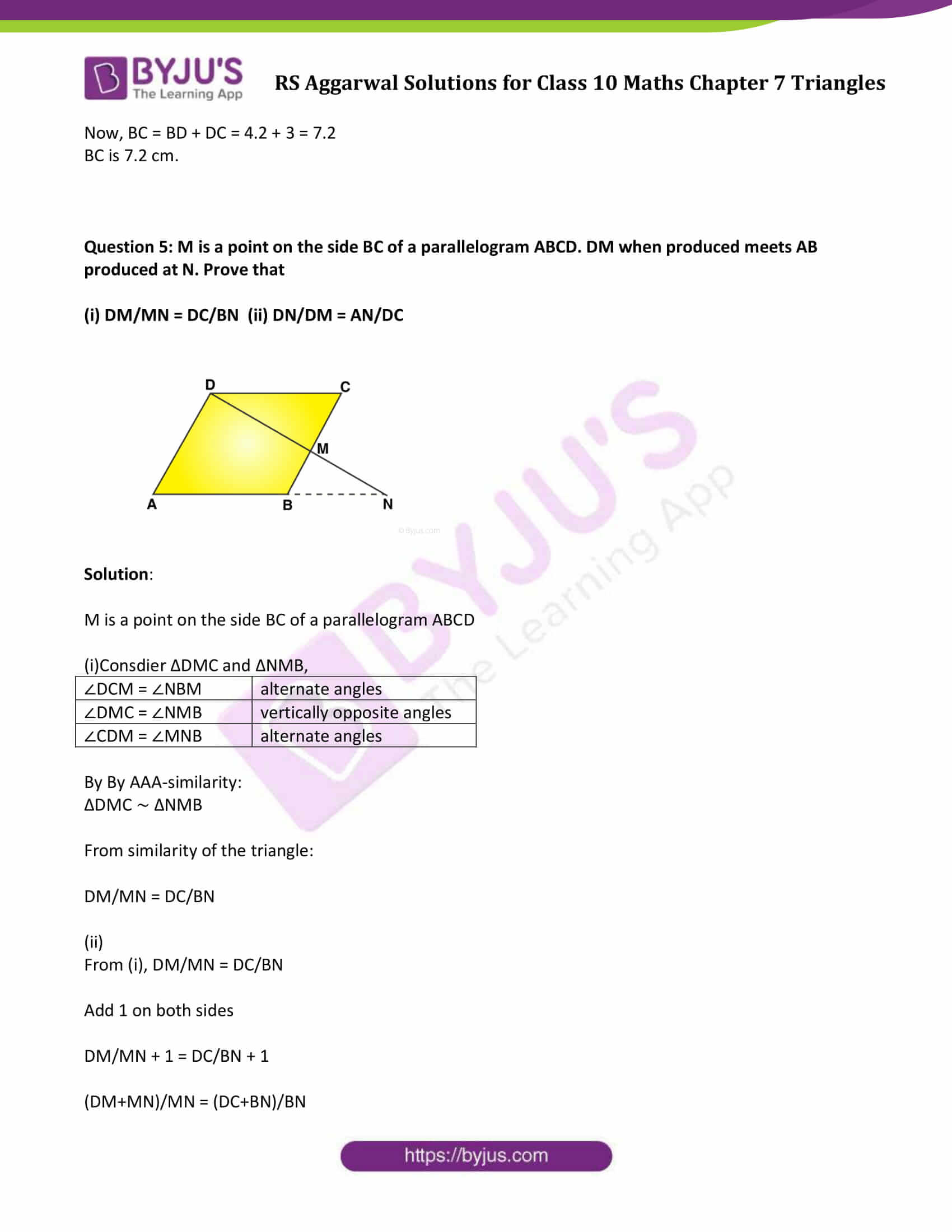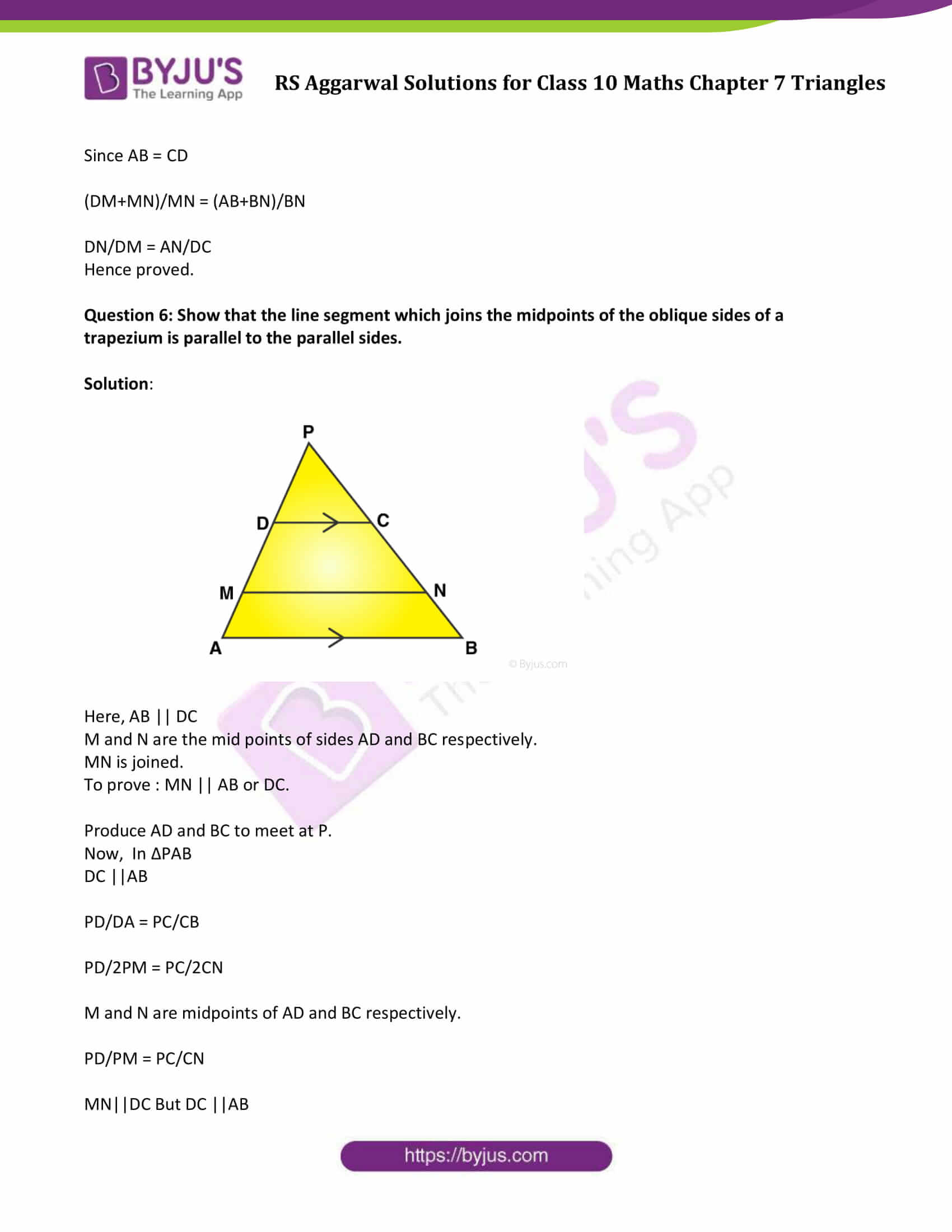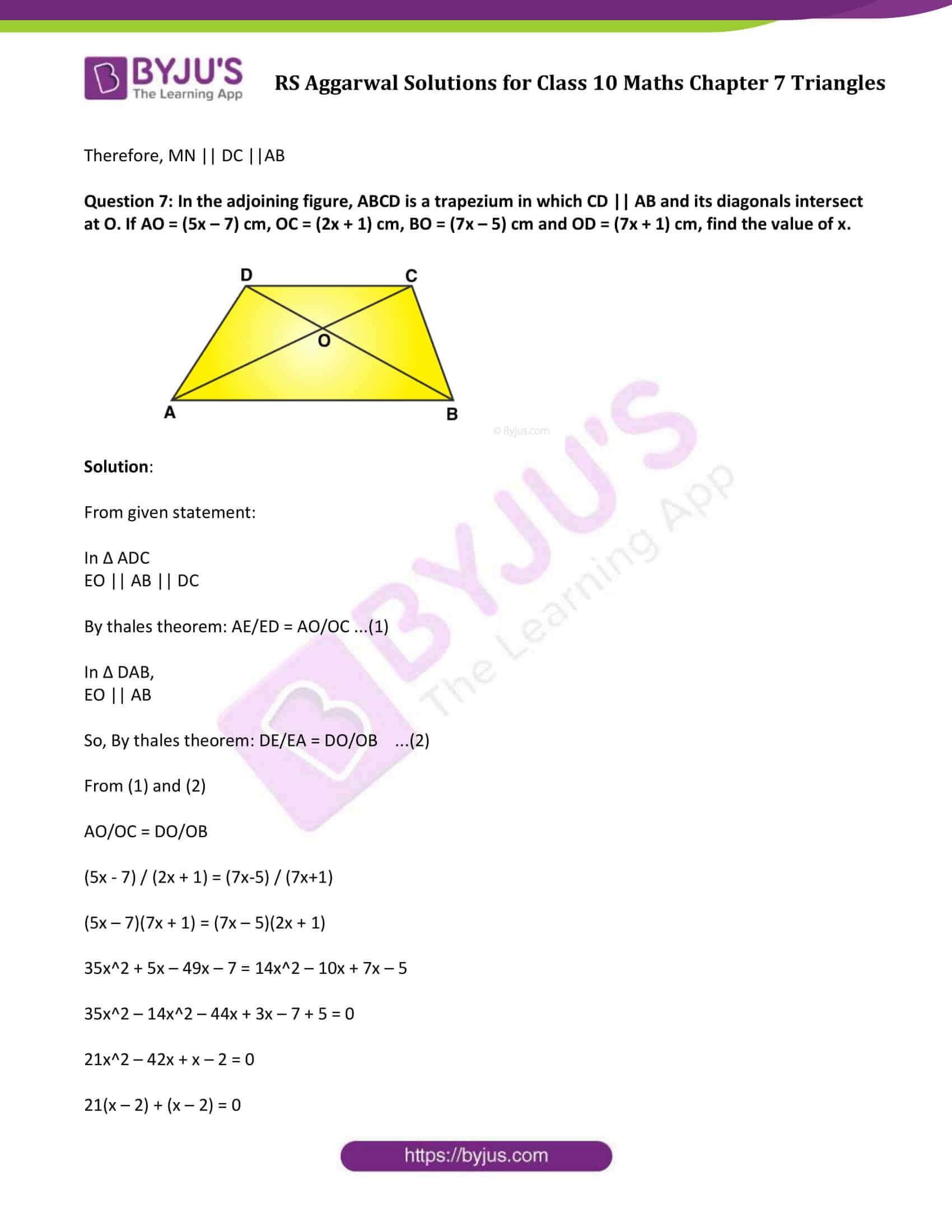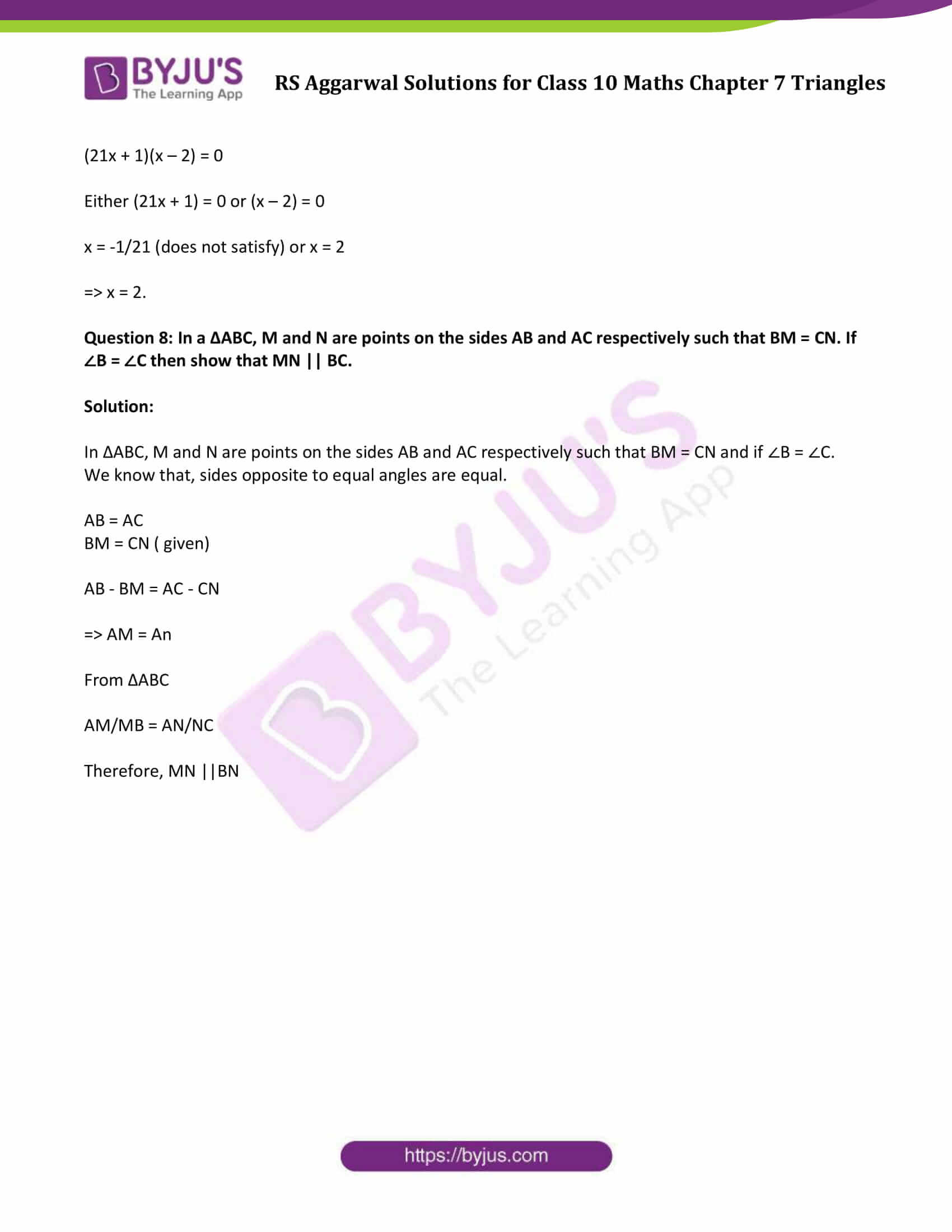### Access other exercise solutions of Class 10 Maths Chapter 7 Triangles

Exercise 7 B Solutions

Exercise 7 C Solutions

Exercise 7 D Solutions

Exercise 7 E Solutions

Question 1: D and E are points on the sides AB and AC respectively of a ∆ABC such that DE || BC.

(i) If AD = 3.6 cm, AB = 10 cm and AE = 4.5 cm, find EC and AC.

(ii) If AB = 13.3 cm, AC = 11.9 cm and EC = 5.1 cm, find AD.

(iii) If AD/DB = 4/7 and AC = 6.6 cm, find AE.

(iv) if AD/AB = 8/15 and EC = 3.5 cm, find AE.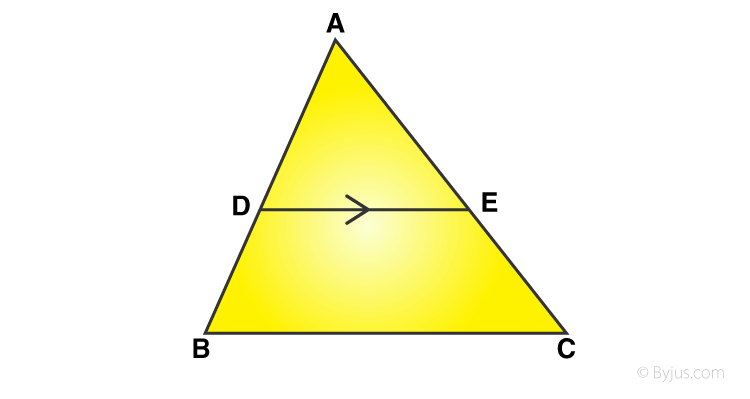Solution:

From given triangle, points D and E are on the sides AB and AC respectively such that DE || BC.

(i)AD = 3.6 cm, AB = 10 cm and AE = 4.5 cm.

By Thale’s Theorem:

Here DB = AB – AD = 10 – 3.6 = 6.4

=> EC = 4.6/3.6 x 6.4

or EC = 8

And, AC = AE + EC

AC = 4.5 + 8 = 12.5

(ii) If AB = 13.3 cm, AC = 11.9 cm and EC = 5.1 cm, find AD.

By Thale’s Theorem:

AD/DB + 1 = AE/EC + 1

(AD + DB)/DB = (AE + EC)/EC

AB/DB = AC/EC

or DB = (ABxEC)/ AC

= (13.3 x 5.1)/11.90

= 5.7

=> BD = 5.7

And, AD = AB – DB

(iii) AD/DB = 4/7 or AD = 4 cm, DB = 7 cm, and AC = 6.6

By Thale’s Theorem:

AD/DB + 1 = AE/EC + 1

(AD + DB)/DB = (AE + EC)/EC

(4+7)/7 = AC/EC = 6.6/EC

EC = (6.6 x 7)/11

= 4.2

And, AE = AC – EC

AE = 6.6 – 4.2

AE = 2.4 cm

(iv)

AD/AB = 8/15 or AD = 8 cm, AB = 15 cm, and EC = 3.5 cm

By Thale’s Theorem:

8/15 = AE/(AE+EC) = AE/(AE+3.5)

8(AE + 3.5) = 15AE

7 AE = 28

or AE = 4 cm

Question 2: D and E are points on the sides AB and AC respectively of a ∆ABC such that DE || BC. Find the value of x, when

(i) AD = x cm, DB = (x – 2) cm, AE = (x + 2) cm and EC = (x – 1) cm.

(ii) AD = 4 cm, DB = (x – 4) cm, AE = 8 cm and EC = (3x – 19) cm.

(iii) AD = (7x – 4) cm, AE = (5x – 2) cm, DB = (3x + 4) and EC = 3x cm.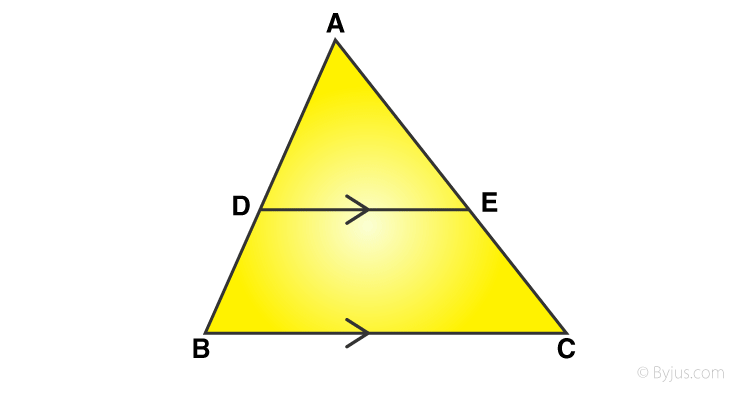Solution:

From figure, D and E are the points on the sides AB and AC respectively and DE || BC

(i) AD = x cm, DB = (x – 2) cm, AE = (x + 2) cm and EC = (x – 1) cm.

x/(x-2) = (x+2)/(x-1)

x(x-1) = (x+2)(x-2)

Solving above equation, we get

x = 4 cm

(ii) AD = 4 cm, DB = (x – 4) cm, AE = 8 cm and EC = (3x – 19) cm.

4/(x-4) = 8/(3x-19)

4(3x-19) = 8(x-4)

Solving, we get x = 11 cm

(iii)

AD = (7x – 4) cm, AE = (5x – 2) cm, DB = (3x + 4) and EC = 3x cm.

(7x-4)/(3x + 4) = (5x – 2)/3x

(7x – 4) (3x) = (5x – 2) (3x + 4)

21x² – 12x – 15x² – 20x + 6x = -8

6x² – 26x + 8 = 0

(x – 4) (3x – 1) = 0

Either x – 4 = 0 or (3x – 1) = 0

=> x = 4 or 1/3 (not possible)

So, x = 4

Question 3: D and E are points on the sides AB and AC respectively of a ∆ABC. In each of the following cases, determine whether DE || BC or not.

(i) AD = 5.7 cm, DB = 9.5 cm, AE = 4.8 cm and EC = 8 cm

(ii) AB = 11.7 cm, AC = 11.2 cm, BD = 6.5 cm and AE = 4.2 cm.

(iii) AB = 10.8 cm, AD = 6.3 cm, AC = 9.6 cm and EC = 4 cm.

(iv) AD = 7.2 cm, AE = 6.4 cm, AB = 12 cm and AC = 10 cm.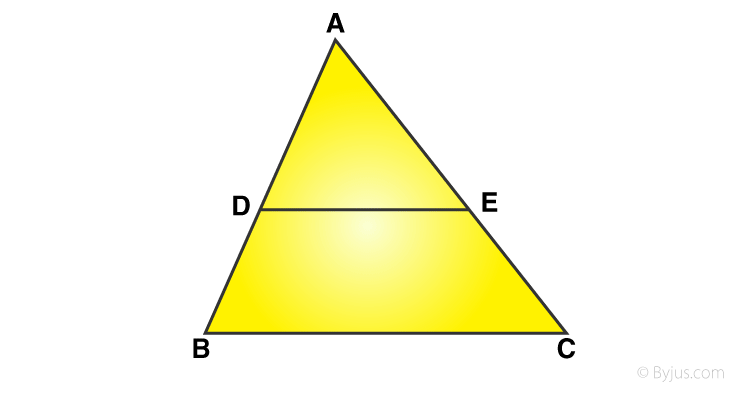Solution:

From figure, D and E are the points on the sides AB and AC of ∆ABC

(i) AD = 5.7 cm, DB = 9.5 cm, AE = 4.8 cm and EC = 8 cm

and AE/EC = 4.8/8 = 3/5

=> DE || BC

(ii) AB = 11.7 cm, AC = 11.2 cm, BD = 6.5 cm and AE = 4.2 cm.

AD = AB – BD = 11.7 – 6.5 = 5.2 cm and

EC = AC – AE = 11.2 – 4.2 = 7 cm

AE/EC = 4.2/7 = 3/5

=> DE is not parallel to BC

(iii) AB = 10.8 cm, AD = 6.3 cm, AC = 9.6 cm and EC = 4 cm.

DB = AB – AD = 10.8 – 6.3 = 4.5 cm

AE =AC – EC = 9.6 – 4 = 5.6 cm

AD /DB = 6.3/4.5 = 7/5

AE/EC = 5.6/4 = 7/5

=> DE || BC

(iv) AD = 7.2 cm, AE = 6.4 cm, AB = 12 cm and AC = 10 cm.

DB = AB – AD = 12 – 7.2 = 4.8 cm and

EC = AC – AE = 10 – 6,4 =3.6 cm

AD /DB = 7.2/4.8 = 3/2 and

AE/EC = 6.4/3.6 = 16/9

=> DE is not parallel to BC

Question 4: In a ∆ABC, AD is the bisector of ∠A.

(i) If AB = 6.4 cm, AC = 8 cm and BD = 5.6 cm, find DC.

(ii) If AB = 10 cm, AC = 14 cm and BC – 6 cm, find BD and DC.

(iii) If AB = 5.6 cm, BD = 3.2 cm and BC = 6 cm, find AC.

(iv) If AB = 5.6 cm, AC = 4 cm and DC = 3 cm, find BC.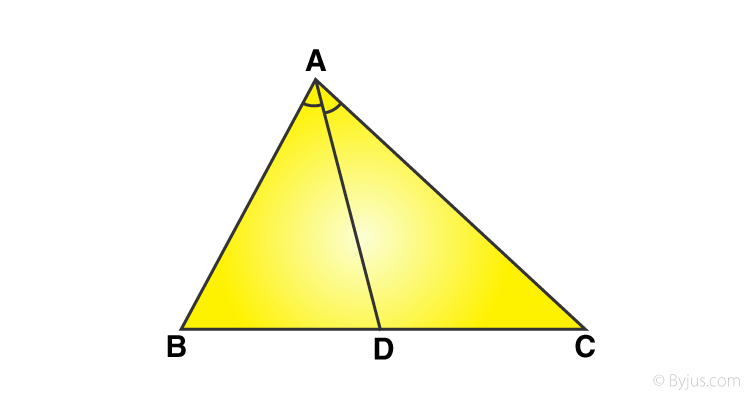Solution:

(i) If AB = 6.4 cm, AC = 8 cm and BD = 5.6 cm, find DC.

AD bisects ∠A, we can apply angle-bisector theorem in ∆ABC,

BD/DC = AB/AC

Substituting given values, we get

5.6/DC = 6.4/8

DC = 7 cm

(ii) If AB = 10 cm, AC = 14 cm and BC – 6 cm, find BD and DC.

By angle-bisector theorem

BD/DC = AB/AC = 10/14

Let BD = x cm and DC = (6-x) (As BC = 6 cm given)

x/(6-x) = 10/14

14x = 10(6 – x)

14x = 60 – 10x

14x + 10x = 60

or x = 2.5

Or BD = 2.5

Then DC = 6 – 2.5 = 3.5 cm

(iii) If AB = 5.6 cm, BD = 3.2 cm and BC = 6 cm, find AC.

BD/DC = AB/AC

Here DC = BC – BD = 6 – 3.2 = 2.8

=> DC = 2.8

3.2/2.8 = 5.6/AC

=> AC = 4.9 cm

(iv) If AB = 5.6 cm, AC = 4 cm and DC = 3 cm, find BC.

BD/DC = AB/AC

BD/3 = 5.6/4

=> BD = 4.2

Now, BC = BD + DC = 4.2 + 3 = 7.2

BC is 7.2 cm.

Question 5: M is a point on the side BC of a parallelogram ABCD. DM when produced meets AB produced at N. Prove that

(i) DM/MN = DC/BN (ii) DN/DM = AN/DC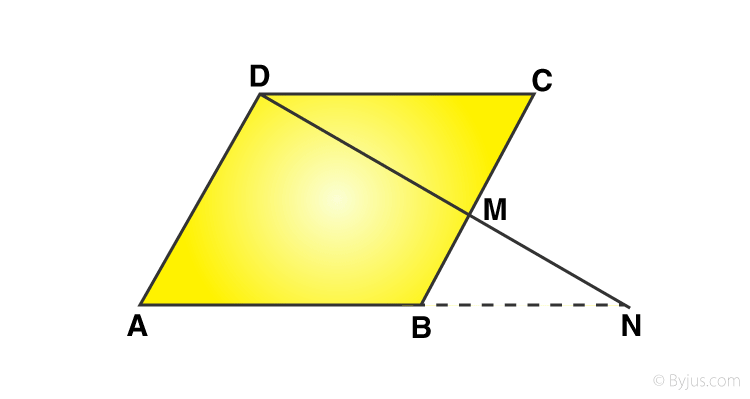Solution:

M is a point on the side BC of a parallelogram ABCD

(i)Consdier ∆DMC and ∆NMB,

 ∠DCM = ∠NBM alternate angles ∠DMC = ∠NMB vertically opposite angles ∠CDM = ∠MNB alternate angles

By By AAA-similarity:

∆DMC ∼ ∆NMB

From similarity of the triangle:

DM/MN = DC/BN

(ii)

From (i), DM/MN = DC/BN

DM/MN + 1 = DC/BN + 1

(DM+MN)/MN = (DC+BN)/BN

Since AB = CD

(DM+MN)/MN = (AB+BN)/BN

DN/DM = AN/DC

Hence proved.

Question 6: Show that the line segment which joins the midpoints of the oblique sides of a trapezium is parallel to the parallel sides.

Solution: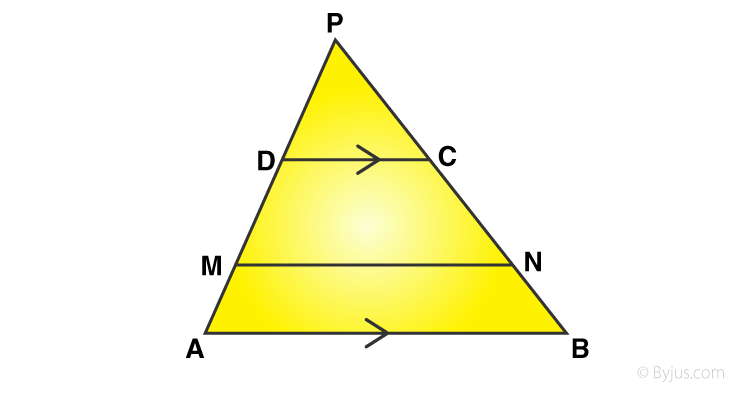Here, AB || DC

M and N are the mid points of sides AD and BC respectively.

MN is joined.

To prove : MN || AB or DC.

Produce AD and BC to meet at P.

Now, In ∆PAB

DC ||AB

PD/DA = PC/CB

PD/2PM = PC/2CN

M and N are midpoints of AD and BC respectively.

PD/PM = PC/CN

MN||DC But DC ||AB

Therefore, MN || DC ||AB

Question 7: In the adjoining figure, ABCD is a trapezium in which CD || AB and its diagonals intersect at O. If AO = (5x – 7) cm, OC = (2x + 1) cm, BO = (7x – 5) cm and OD = (7x + 1) cm, find the value of x.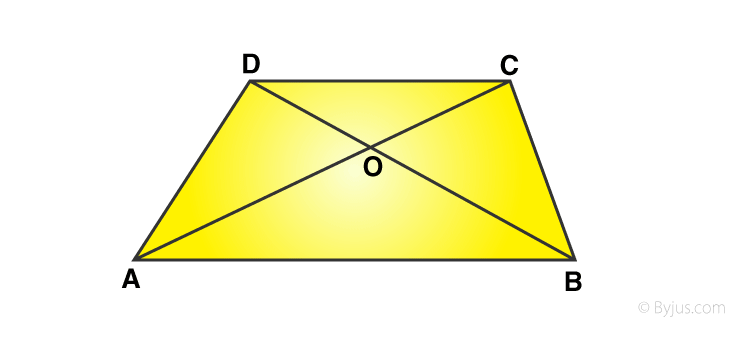Solution:

From given statement:

EO || AB || DC

By thales theorem: AE/ED = AO/OC …(1)

In Δ DAB,

EO || AB

So, By thales theorem: DE/EA = DO/OB …(2)

From (1) and (2)

AO/OC = DO/OB

(5x – 7) / (2x + 1) = (7x-5) / (7x+1)

(5x – 7)(7x + 1) = (7x – 5)(2x + 1)

35x^2 + 5x – 49x – 7 = 14x^2 – 10x + 7x – 5

35x^2 – 14x^2 – 44x + 3x – 7 + 5 = 0

21x^2 – 42x + x – 2 = 0

21(x – 2) + (x – 2) = 0

(21x + 1)(x – 2) = 0

Either (21x + 1) = 0 or (x – 2) = 0

x = -1/21 (does not satisfy) or x = 2

=> x = 2.

Question 8: In a ∆ABC, M and N are points on the sides AB and AC respectively such that BM = CN. If ∠B = ∠C then show that MN || BC.

Solution:

In ∆ABC, M and N are points on the sides AB and AC respectively such that BM = CN and if ∠B = ∠C.

We know that, sides opposite to equal angles are equal.

AB = AC

BM = CN ( given)

AB – BM = AC – CN

=> AM = An

From ∆ABC

AM/MB = AN/NC

Therefore, MN ||BN

## RS Aggarwal Solutions for Class 10 Maths Chapter 7 Triangles Exercise 7A

Class 10 Maths Chapter 7 Triangles Exercise 7A is based on the following topics and subtopics:

• Congruent figures
• Similar figures
• Similar Polygons
• Equiangular triangles
• Similar Triangles
• Important Theorems on Triangles:
• Thales’ Theorem and it’s converse
• Midpoint theorem
• Angle-Bisector Theorem